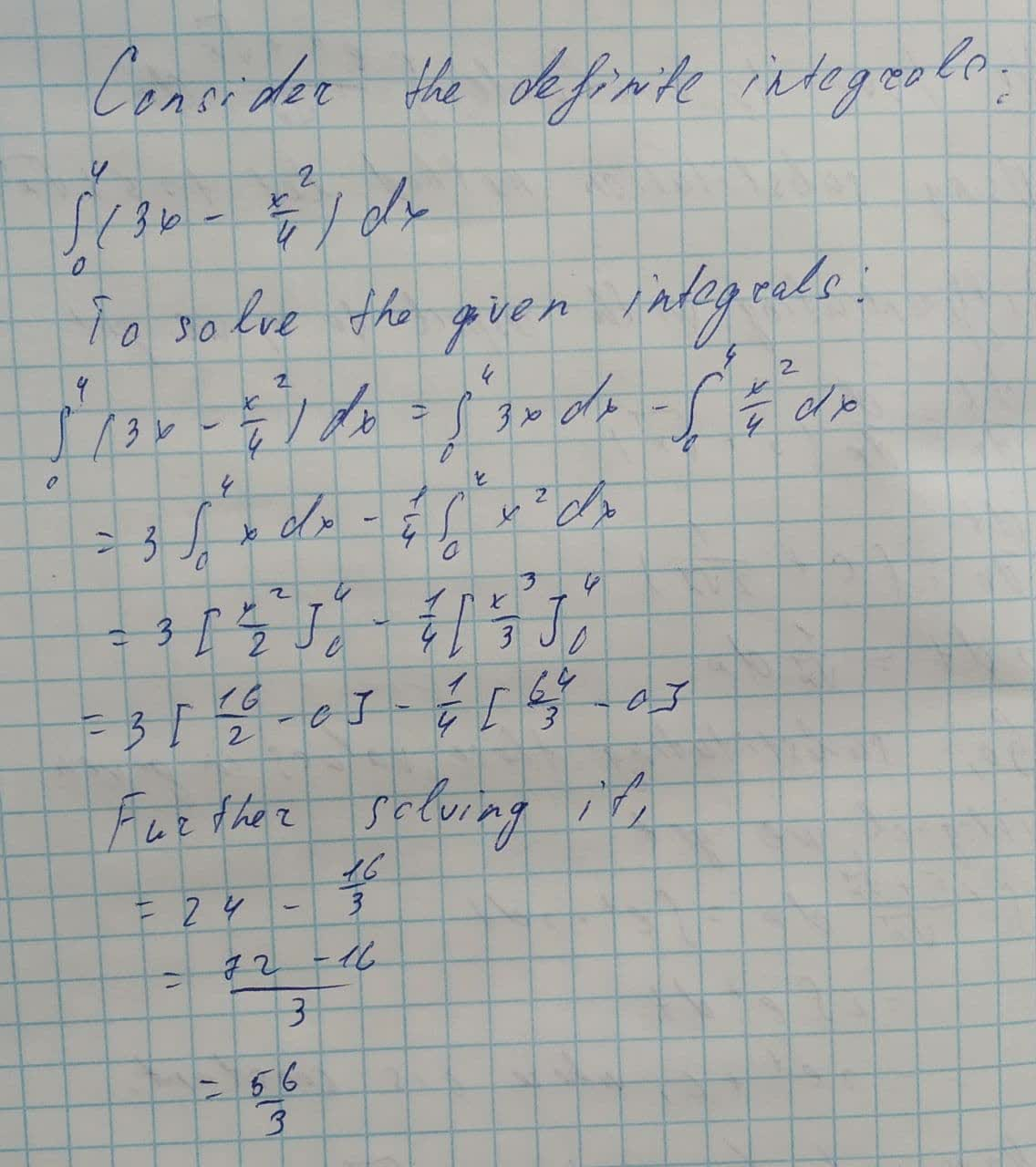Question# Evaluate the definite integral \int_{0}^{4}(3x-\frac{x^{2}}{4})dx

Applications of integrals
ANSWEREDEvaluate the definite integral.
$$\displaystyle{\int_{{{0}}}^{{{4}}}}{\left({3}{x}-{\frac{{{x}^{{{2}}}}}{{{4}}}}\right)}{\left.{d}{x}\right.}$$